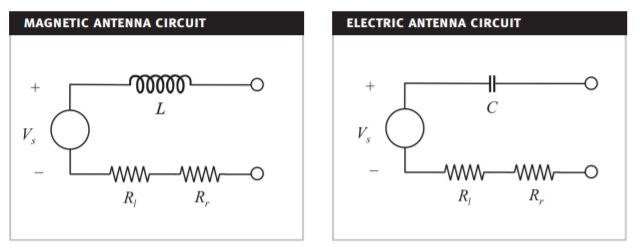# 5. Circuit Modeling¶

The operation of the antenna can be analyzed by using an equivalent circuit. The ferrite rod antenna consists of a coil which can be modeled as an inductor that has various resistances in series due to the antenna losses. Together with a parallel capacitance the antenna forms a parallel RLC circuit. The RLC resonance frequency can be tuned by adjusting the capacitance of the capacitor. The impedance seen from the antenna terminals is the antenna impedance [Koskimaa, 2016]:

(5.1)$Z_A = R_A + jX_A$Fig. 5.1 : Circuit models of magnetic and electric antennas.